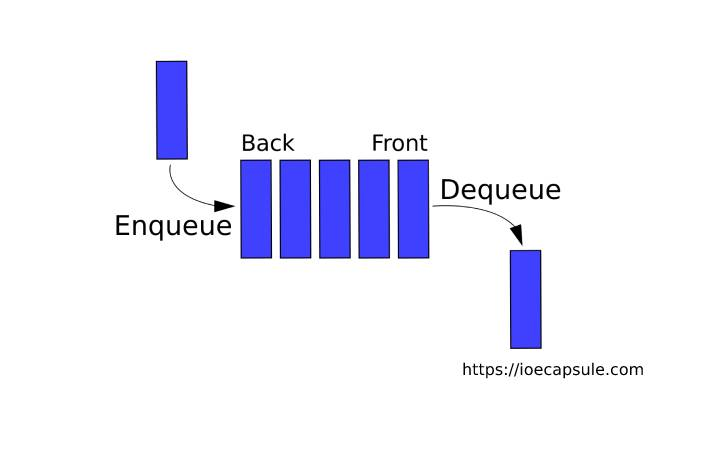### Queue : Linked List Implementation Using C++Data Structures

A Queue is a linear, ordered collection of items or data structure in which the operations are performed in First In First Out fashion . Meaning, it is always the first item to be put into the queue, and first item is to be removed first .

#### Linked List Implementation Of Queue Using C++

``````
#include<iostream>
using namespace std;

template<typename T> class Node{
private:
T data;
Node<T>* next;
template<typename U>friend class Queue;
};

template<typename T> class Queue{
private:
Node<T>* first;
public:
Queue(){
first = NULL;
}

bool empty(){
return first == NULL;
}

void enqueue(T item){
Node<T>* node = new Node<T>;
node->data = item;
if(empty()){
first = node;
}else{
Node<T>* temp = first;
while(temp->next != NULL){
temp = temp->next;
}
temp->next = node;
}
cout<<item<<" enqueued"<<endl;
}

void dequeue(){
if(empty()){
cout<<"queue is empty"<<endl;
return;
}
T item = first->data;
first = first->next;
cout<<item<<" dequeued"<<endl;
}

T front(){
if(empty()){
return NULL;
}
return this->first->data;
}
};

int main(){
bool quit = false;
Queue<int> queue;
int temp;
do{
cout<<"===================================="<<endl;
cout<<"select option :"<<endl;
cout<<"1 for enqueue"<<endl;
cout<<"2 for dequeue"<<endl;
cout<<"3 for front item"<<endl;
cout<<"4 for exit"<<endl;
int ch;
cin>>ch;
cout<<"===================================="<<endl;
switch (ch)
{
case 1:
cout<<"enter item to enqueue:"<<endl;
cin>>temp;
queue.enqueue(temp);
break;
case 2:
queue.dequeue();
break;
case 3:
if(queue.empty()){
cout<<"queue is empty"<<endl;
}else{
cout<<"front: "<<queue.front()<<endl;
}
break;
case 4:
quit = true;
break;
default:
cout<<"invalid selection"<<endl;
break;
}
}while(!quit);
return 0;
}``````